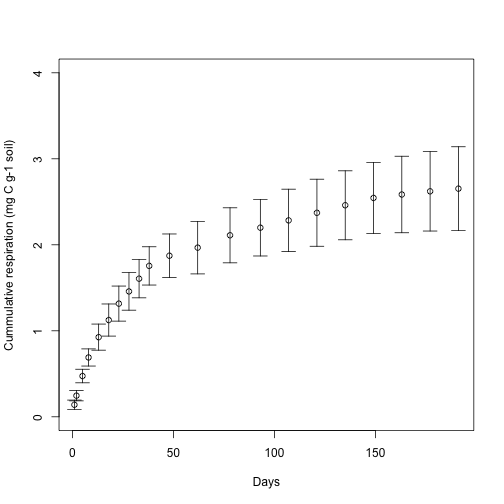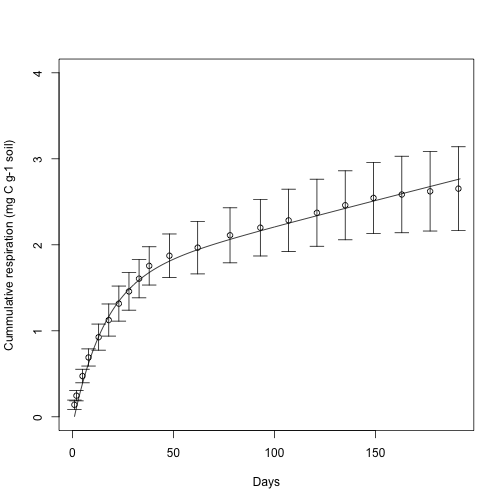SoilR, in combination with another optimization package such as FME, can assist you in identifying kinetic soil organic matter fractions through the process of parameter estimation. The procedure consists of: 1) prepare a dataset of observed CO2 fluxes from a soil incubation experiment, 2) setting up a model that can potentially explain the data, 3) create a cost function to optimize, 4) perform the optimization.

For this example, we will use data from an incubation experiment performed by Dr. Susan E. Crow at the University of Hawaii using a soil from a bare agricultural field. For the optimization we will use R package FME.

## 1. Preparation of the dataset

The dataset contains observations of CO2 efflux from soil incubations carried out in 2010 during 191 days. The data is presented as cummulative CO2 and the average of 4 replicates.

CO2flux=data.frame(
time=c(1,2,5,8,13,18,23,28,33,38,48,62,78,93,107,121,135,149,163,177,191),
cummCO2=c(0.140,0.246,0.474,0.690,0.926,1.125,1.316,1.458,1.606,1.755,1.873,
1.967,2.111,2.199,2.284,2.372,2.460,2.545,2.585,2.622,2.653),
Stderr=c(0.054,0.060,0.078,0.100,0.152,0.187,0.204,0.219,0.222,0.223,0.253,
0.305,0.320,0.329,0.362,0.390,0.401,0.413,0.444,0.462,0.488)
)


The initial amount of C at the begining of the incubation is

Ctotal=20.0 #C concentration in soil sample (mg C g-1 soil)


A plot of the data

plot(CO2flux[,1:2],type="p",xlab="Days",
ylab="Cummulative respiration (mg C g-1 soil)",  ylim=c(0,4))
arrows(CO2flux[,1],CO2flux[,2]-CO2flux[,3],CO2flux[,1],CO2flux[,2]+
CO2flux[,3],angle=90, length=0.1,code=3)## 2. Setting up the model

For this example we will set up a 2-pool model with feedback of the form

$\left( \begin{array}{c} \frac{dC_1}{dt} \\ \frac{dC_2}{dt} \end{array} \right) = \left( \begin{array}{cc} -k_1 & \alpha_{1,2} k_2 \\ \alpha_{2,1} k_1 & -k_2 \end{array} \right) \cdot \left( \begin{array}{c} C_1 \\ C_2 \end{array} \right)$

with initial conditions

$\left( \begin{array}{c} C_1(t= 0) = \gamma_1 C_{total} \\ C_2(t=0) = (1-\gamma_1) C_{total} \end{array} \right)$

For this example, we will identify the decomposition rates k, the transfer coefficients alpha, and the fractionation coefficient gamma.

We now load the required SoilR and FME packages

library(SoilR)
library(FME)

## Loading required package: rootSolve

## Loading required package: coda


and create a vector of time points to solve the model for these time points

days=seq(0,191) #Incubation days


Lastly, we set up the model using the function TwopFeedbackModel to produce output that resembles the observed data

eCO2func=function(pars){
mod=TwopFeedbackModel(
t=days,
ks=pars[1:2],
a21=pars*pars,
a12=pars*pars,
C0=Ctotal*c(pars,1-pars),
In=0,
pass=TRUE
)
AccR=getAccumulatedRelease(mod)
return(data.frame(time=days,cummCO2=rowSums(AccR)))
}


## 3. Create a cost/objective function

We now create a cost function that compares predictions and observations. This function will be used by the optimization algorithm to minimize the difference between predictions and observations. FME function modCost can create the cost function automatically, and I strongly recommend you to look at the help of this function using the command ?modCost. For our example we create this cost function as

eCO2cost=function(pars){
modelOutput=eCO2func(pars)
return(modCost(model=modelOutput, obs=CO2flux, err="Stderr"))
}


This cost function takes any set of parameter values, runs the model, and calculates the difference between predictions and observations using the reported standard errors to calculated weighed residuals.

## 4. Fit the model through optimization

We can now run an optimization of the cost function given an initial set of parameter values

inipars=c(k1=0.5,k2=0.05,alpha21=0.5,alpha12=0.1,gamma=0.5)


This initial set of parameters tells the optimization algorithm where to start the search. In case of poor convergance, you can change these initial values.

To run the optimization, we use the function modFit from FME as

eCO2fit=modFit(f=eCO2cost,p=inipars,method="Nelder-Mead",
upper=c(Inf,Inf,1,1,1),lower=c(0,0,0,0,0))


Notice that we give lower bounds to all parameters and upper bounds to the transfer and fractionation coefficients. For this example we used the Nelder-Mead optimization algorithm, but you can choose between different methods. Details can be found in the help of the function ?modFit. The best set of parameter values can be retrieved as

eCO2fit$par  ## k1 k2 alpha21 alpha12 gamma ## 0.0644160343 0.0003811812 0.8918784332 0.1027636061 0.7700674412  We can now run the model again with the best parameter set and visually compare the fit #Run the model again with best parameter set fitmod=TwopFeedbackModel(t=days, ks=eCO2fit$par[1:2],
a21=eCO2fit$par*eCO2fit$par,
a12=eCO2fit$par*eCO2fit$par,
C0=Ctotal*c(eCO2fit$par,1-eCO2fit$par),
In=0)
fitCum=getAccumulatedRelease(fitmod)

#Plot the results
plot(CO2flux[,1:2],type="p",xlab="Days",
ylab="Cummulative respiration (mg C g-1 soil)", ylim=c(0,4))
arrows(CO2flux[,1],CO2flux[,2]-CO2flux[,3],CO2flux[,1],CO2flux[,2]+
CO2flux[,3],angle=90, length=0.1,code=3)
lines(rowSums(fitCum))You can also continue the analysis further and perform a Bayesian optimization. For details see the vignettes in the FME and SoilR packages.

## Summary

With this procedure we were able to obtain 5 parameter values using only CO2 efflux and total initial carbon data from an incubation experiment. The procedure showed that one kinetic pool cycles at a rate of 0.06 per day, and the other pool cycles much slower at a rate of 0.0004 per day. The transfer coefficients also tell us that about 90% of the C cycled in the fast pool is transferred to the slow pool, and the slow pool feedbacks about 10% of the carbon processed there.

We can also infer that from the initial amount of C at the beginin of the incubation, 77% was stored in the fast cycling pool, while the remaining 33% was stored in the slow cycling pool. This procedure therefore, help us to determine the size of meaningful kinetic pools without resorting to physical or chemical fractionations.